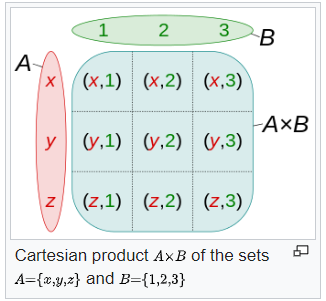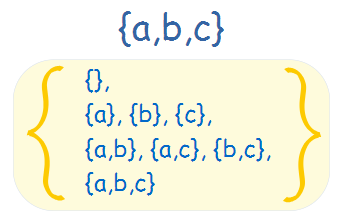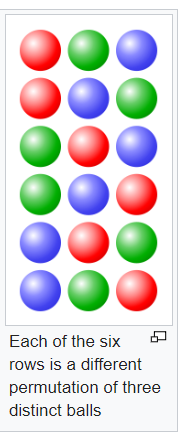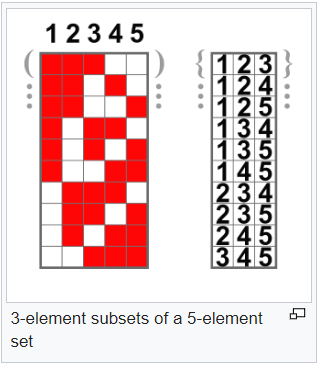# Python Itertools: For a faster and memory efficient code

The reason python stands out from many other languages is because of it’s simplicity and easy to work with, and the data science community has put the work in to create the plumbing it needs to solve complex computational problems and emphasizes productivity and readability. However many complains that it’s slow and doesn’t perform very well on a large set of data. There are lot of effective and memory efficient python modules which are not well known among the community and especially beginners doesn’t learn those things in MOOC’s. Since MOOC’s is a way to introduce you with the python ecosystem but it doesn’t tells you how you can write concise, fast and performance oriented codes. Even many of the experienced dev doesn’t use the power of these awesome python tools,functions etc. Most of them try to achieve the things in the same old memory inefficient way.

As a Data Scientist, When you are working with millions of records then you understand how important it is to have faster processing of your data and importance of memory efficient codes. Python provides lot of options to write a memory efficient code and do the things in a simpler way. In this post I am trying to cover some of those techniques which you can leverage in your daily life and convert your codes into much pythonic way and can shout Python is Awesome and it runs faster than your expectations

What is an Iterator?

iterator is an object which can be iterated upon and returns one item at a time and it consists of two dunder methods iter() and next() which is known as iterators protocol. All the iterator objects like List, Tuples, Dictionary have built-in iter method which is used to get an iterator and the next method gives the next item in the sequence when you want to iterate manually. You have seen for loop is used to iterate through any of these iterables.

Python provides an excellent module to handle the iterators and that is called as itertools. As per the Python Documentation:

The module standardizes a core set of fast, memory efficient tools that are useful by themselves or in combination. Together, they form an “iterator algebra” making it possible to construct specialized tools succinctly and efficiently in pure Python

Here are some of the examples on how to use the itertools in your code:

Product

Find Cartesian Product of these three lists:

``````A = ['a','b','c']
B = [1, 2]
C=['p','q','r']
``````

what is Cartesian Product?source: wikipedia

Solution:

``````import itertools
for element in itertools.product(A,B,C,repeat=1):
print(element)
``````

Cartesian Product of the above three list :

(‘a’, 1, ‘p’), (‘a’, 1, ‘q’), (‘a’, 1, ‘r’), (‘a’, 2, ‘p’), (‘a’, 2, ‘q’), (‘a’, 2, ‘r’), (‘b’, 1, ‘p’), (‘b’, 1, ‘q’), (‘b’, 1, ‘r’), (‘b’, 2, ‘p’), (‘b’, 2, ‘q’), (‘b’, 2, ‘r’), (‘c’, 1, ‘p’), (‘c’, 1, ‘q’), (‘c’, 1, ‘r’), (‘c’, 2, ‘p’), (‘c’, 2, ‘q’), (‘c’, 2, ‘r’)

Groupby

It is a tool for grouping items . groupby objects yield key-group pairs where the group is a generator.

Features

• Group consecutive items together
• Group all occurrences of an item, given a sorted iterable
• Specify how to group items with a key function

Find the Key of different group in this String:

AAAABBBCCDAABBB

Solution:

``````[k for k, g in groupby(''AAAABBBCCDAABBB ")]
``````

Result:

A B C D A B

Another Example, Find length of each items in the above String:

Solution:

``````[(c,len(list(cgen))) for c,cgen in groupby( 'AAAABBBCCDAABBB' )]
``````

Result:

(‘A’, 4), (‘B’, 3), (‘C’, 2), (‘D’, 1), (‘A’, 2), (‘B’, 3)

Accumulate

The accumulate iterator returns the accumulated sums or the accumulated results of a two argument function that you can pass to accumulate. The default of accumulate is addition

Find the product of two items in this list:

``````data = [3, 4, 6, 2, 1, 9, 0, 7, 5, 8]
``````

Solution:

`````` list(accumulate(data, operator.mul))
``````

Expected Result:

``````[3, 12, 72, 144, 144, 1296, 0, 0, 0, 0]

i.e. [3,4x3,4x3x6,4x3x6x2,4x3x6x2x1, 4x3x6x2x1x9,
4x3x6x2x1x9 x0,0x7,0x5,0x8 ]
``````

Dropwhile and takewhile

As per it’s Documentation, it Make an iterator that drops elements from the iterable as long as the predicate is true; afterwards, returns every element. The iterator does not produce any output until the predicate first becomes false, so it may have a lengthy start-up time

Drop all values to get a list of all zeros in this list

``````l= [0.01, 0.02, 0.01, -0.01, 0, 0, 0, 0, 0, 0, 0, 0, 0]
``````

Solution:

``````list(itertools.dropwhile(lambda x: x != 0, l))
``````

Result:

``````[ 0, 0, 0, 0, 0, 0, 0, 0, 0 ]
``````

This expression drop all elements in the list until first zero is not encountered

Another Example, Drop all values to get a list of Non-Zeros in the above list

Solution:

`````` list(itertools.takewhile(lambda x: x != 0, l))
``````

Result:

``````[0.01, 0.02, 0.01, -0.01]
``````

This expression takes all elements in the list until first zero is not encountered

Chains

Flatten this list:

``````l = [[1, 2, 3], [4, 5, 6], , [8, 9]]
``````

Solution:

``````list(itertools.chain(*list2d))
``````

Result:

``````[1,2,3,4,5,6,7,8,9]
``````

Chain.fromiterables

The chain method takes 0 or more arguments, each an iterable, Chain.fromiterables takes one argument which is expected to produce the iterables

Find Powerset of a this list elements:

``````[1,2,3]
``````

What is Powerset?Solution:

``````s = list(iterable)
for item in chain.from_iterable(combinations(s, r) for r in range(len(s)+1)):
print(item
``````

Result:

``````powerset([1,2,3]) --> () (1,) (2,) (3,) (1,2) (1,3) (2,3) (1,2,3)
``````

Permuations

It takes an arbitrary iterable as an argument, and always returns an iterator yielding tuplessource: wikipedia

Find all Permuations of the items in this list:

``````[0, 1, 2,]
``````

Solution:

``````obj = itertools.permutations(range(3))
print(list(obj))
``````

Result:

``````[(0, 1, 2), (0, 2, 1), (1, 0, 2), (1, 2, 0), (2, 0, 1), (2, 1, 0)]
``````

Compress:

It allows you to filters elements, returning only those that have a corresponding element that evaluates to True

Find the words in the below words list which has value in counts list:

``````words = ['hello', 'how', 'are', 'you']
counts = [10, '', 'x', None]
``````

Solution:

`````` list(compress(words, counts))
``````

Result:

``````['hello', 'are']
``````

Combinations

As per Wiki, a combination is a selection of items from a collection, such that the order of selection does not matter. For example, given three fruits, say an apple, an orange and a pear, there are three combinations of two that can be drawn from this set: an apple and a pear; an apple and an orange; or a pear and an orange.source: Wikipedia

Find all possible combinations for this list:

``````lst = [1, 2, 3]
``````

Solution:

``````for i in xrange(1, len(lst)+1):
combs.append(i)
els = [list(x) for x in itertools.combinations(lst, i)]
combs.append(els)
``````

Result:

``````[1, [, , ], 2, [[1, 2], [1, 3], [2, 3]], 3, [[1, 2, 3]]]
``````

Similarly you can use combinations_with_replacement method allowing individual elements to be repeated more than once.

islice

As per the Documentation, it makes an iterator that returns selected elements from the iterable. If start is non-zero, then elements from the iterable are skipped until start is reached. Afterward, elements are returned consecutively unless step is set higher than one which results in items being skipped. If stop is `None`, then iteration continues until the iterator is exhausted, if at all; otherwise, it stops at the specified position. Unlike regular slicing, `islice()` does not support negative values for startstop, or step. Can be used to extract related fields from data where the internal structure has been flattened

Slice the first two elements from this list

``````[10, 20, 30, 40, 50, 60]
``````

Solution:

``````for item in itertools.islice(lst,2):
print(item)
``````

Another Example, Find all characters starting from 0 and in step of 2

``````islice('ABCDEFG', 0, None, 2)
``````

Result:

A C E G

islice returns an iterator and thats the man difference between a normal slicing and islice that `islice` doesn’t create a new list, whereas regular list slicing does.

Conclusion:

We have seen how useful and easy it is to use itertools module and it can do lot of work under the hood in a more memory efficient way. As a Data Scientist where you have to crunch million of records and iterate thru it to accomplish your task these handy solutions come as a boon. There are other itertools methods which you can find in the Python official documentation.

I would love to hear your feedback on this article and if you find it useful or want me to improve something please drop me a note in the comments section or write to me at vinaybabu2909@gmail.com

Tags:

Categories:

Updated: